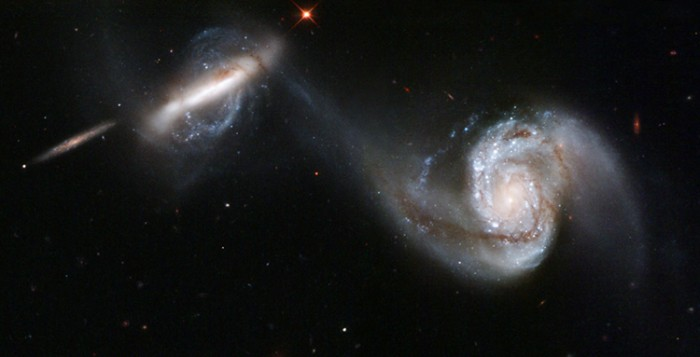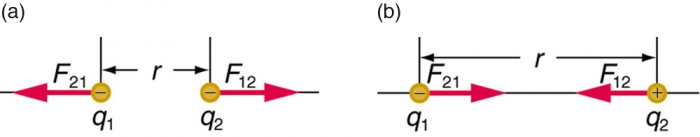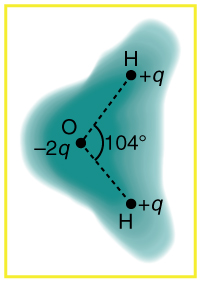## Coulomb’s Law

### Learning Objectives

By the end of this section, you will be able to:

• State Coulomb’s law in terms of how the electrostatic force changes with the distance between two objects.
• Calculate the electrostatic force between two charged point forces, such as electrons or protons.
• Compare the electrostatic force to the gravitational attraction for a proton and an electron; for a human and the Earth.Figure 1. This NASA image of Arp 87 shows the result of a strong gravitational attraction between two galaxies. In contrast, at the subatomic level, the electrostatic attraction between two objects, such as an electron and a proton, is far greater than their mutual attraction due to gravity. (credit: NASA/HST)

Through the work of scientists in the late 18th century, the main features of the electrostatic force—the existence of two types of charge, the observation that like charges repel, unlike charges attract, and the decrease of force with distance—were eventually refined, and expressed as a mathematical formula. The mathematical formula for the electrostatic force is called Coulomb’s law after the French physicist Charles Coulomb (1736–1806), who performed experiments and first proposed a formula to calculate it.

### Coulomb’s Law

$\displaystyle{F}=k\frac{\mid{q}_1q_2\mid}{r^2}\\$

Coulomb’s law calculates the magnitude of the force F between two point charges, q1 and q2, separated by a distance r. In SI units, the constant k is equal to

$\displaystyle{k}=8.988\times10^9\frac{\text{N}\cdot\text{m}^2}{\text{C}^2}\approx8.99\times10^9\frac{\text{N}\cdot\text{m}^2}{\text{C}^2}\\$

The electrostatic force is a vector quantity and is expressed in units of newtons. The force is understood to be along the line joining the two charges. (See Figure 2).

Although the formula for Coulomb’s law is simple, it was no mean task to prove it. The experiments Coulomb did, with the primitive equipment then available, were difficult. Modern experiments have verified Coulomb’s law to great precision. For example, it has been shown that the force is inversely proportional to distance between two objects squared $\left(F\propto\frac{1}{r^2}\right)\\$ to an accuracy of 1 part in 1016. No exceptions have ever been found, even at the small distances within the atom.Figure 2. The magnitude of the electrostatic force F between point charges q1 and q2 separated by a distance r is given by Coulomb’s law. Note that Newton’s third law (every force exerted creates an equal and opposite force) applies as usual—the force on q1 is equal in magnitude and opposite in direction to the force it exerts on q2. (a) Like charges. (b) Unlike charges.

### Example 1. How Strong is the Coulomb Force Relative to the Gravitational Force?

Compare the electrostatic force between an electron and proton separated by 0.530 × 10−10 m with the gravitational force between them. This distance is their average separation in a hydrogen atom.

#### Strategy

To compare the two forces, we first compute the electrostatic force using Coulomb’s law, $\displaystyle{F}=k\frac{\mid{q}_1q_2\mid}{r^2}\\$. We then calculate the gravitational force using Newton’s universal law of gravitation. Finally, we take a ratio to see how the forces compare in magnitude.

#### Solution

Entering the given and known information about the charges and separation of the electron and proton into the expression of Coulomb’s law yields

$\begin{array}{lll}{F}&=&k\frac{\mid{q}_1q_2\mid}{r^2}\\\text{ }&=&8.99\times10^9\frac{\text{N}\cdot\text{m}^2}{\text{C}^2}\times\frac{\left(1.60\times10^{-19}\text{ C}\right)\left(1.60\times10^{-19}\text{ C}\right)}{\left(0.530\times10^{-10}\text{ m}\right)^2}\end{array}\\$

Thus the Coulomb force is F = 8.19 × 10−8 N.

The charges are opposite in sign, so this is an attractive force. This is a very large force for an electron—it would cause an acceleration of 8.99 × 1022 m/s2 (verification is left as an end-of-section problem).The gravitational force is given by Newton’s law of gravitation as:

$F_G=G\frac{mM}{r^2}\\$,

where G = 6.67 × 10−11 N · m2/kg2. Here m and M represent the electron and proton masses, which can be found in the appendices. Entering values for the knowns yields

$F_G=\left(6.67\times10^{-11}\text{N}\cdot\text{m}^2\text{/kg}^2\right)\times\frac{\left(9.11\times10^{-31}\text{ kg}\right)\left(1.67\times10^{-27}\text{ kg}\right)}{\left(0.530\times10^{-10}\text{ m}\right)^2}=3.61\times10^{-47}\text{ N}\\$

This is also an attractive force, although it is traditionally shown as positive since gravitational force is always attractive. The ratio of the magnitude of the electrostatic force to gravitational force in this case is, thus, $\frac{F}{F_G}=2.27\times10^{39}\\$.

#### Discussion

This is a remarkably large ratio! Note that this will be the ratio of electrostatic force to gravitational force for an electron and a proton at any distance (taking the ratio before entering numerical values shows that the distance cancels). This ratio gives some indication of just how much larger the Coulomb force is than the gravitational force between two of the most common particles in nature.

As the example implies, gravitational force is completely negligible on a small scale, where the interactions of individual charged particles are important. On a large scale, such as between the Earth and a person, the reverse is true. Most objects are nearly electrically neutral, and so attractive and repulsive Coulomb forces nearly cancel. Gravitational force on a large scale dominates interactions between large objects because it is always attractive, while Coulomb forces tend to cancel.

## Section Summary

• Frenchman Charles Coulomb was the first to publish the mathematical equation that describes the electrostatic force between two objects.
• Coulomb’s law gives the magnitude of the force between point charges. It is $F=k\frac{\mid{q}_{1}{q}_{2}\mid}{{r}^{2}}\\$, where q1 and q2 are two point charges separated by a distance r, and $k\approx8.99\times{10}^{9}\frac{\text{N}\cdot {\text{m}}^{2}}{{\text{C}}^{2}}\\$
• This Coulomb force is extremely basic, since most charges are due to point-like particles. It is responsible for all electrostatic effects and underlies most macroscopic forces.
• The Coulomb force is extraordinarily strong compared with the gravitational force, another basic force—but unlike gravitational force it can cancel, since it can be either attractive or repulsive.
• The electrostatic force between two subatomic particles is far greater than the gravitational force between the same two particles.

### Conceptual QuestionsFigure 3. Schematic representation of the outer electron cloud of a neutral water molecule.

Use Figure 3 as a reference in the following questions. Figure 3 shows a schematic representation of the outer electron cloud of a neutral water molecule. The electrons spend more time near the oxygen than the hydrogens, giving a permanent charge separation as shown. Water is thus a polar molecule. It is more easily affected by electrostatic forces than molecules with uniform charge distributions.

1. Figure 3 shows the charge distribution in a water molecule, which is called a polar molecule because it has an inherent separation of charge. Given water’s polar character, explain what effect humidity has on removing excess charge from objects.
2. Using Figure 3, explain, in terms of Coulomb’s law, why a polar molecule (such as in Figure 3) is attracted by both positive and negative charges.
3. Given the polar character of water molecules, explain how ions in the air form nucleation centers for rain droplets.

### Problems & Exercises

1. What is the repulsive force between two pith balls that are 8.00 cm apart and have equal charges of –30.0 nC?
2. (a) How strong is the attractive force between a glass rod with a 0.700 μC charge and a silk cloth with a –0.600 μC charge, which are 12.0 cm apart, using the approximation that they act like point charges? (b) Discuss how the answer to this problem might be affected if the charges are distributed over some area and do not act like point charges.
3. Two point charges exert a 5.00 N force on each other. What will the force become if the distance between them is increased by a factor of three?
4. Two point charges are brought closer together, increasing the force between them by a factor of 25. By what factor was their separation decreased?
5. How far apart must two point charges of 75.0 nC (typical of static electricity) be to have a force of 1.00 N between them?
6. If two equal charges each of 1 C each are separated in air by a distance of 1 km, what is the magnitude of the force acting between them? You will see that even at a distance as large as 1 km, the repulsive force is substantial because 1 C is a very significant amount of charge.
7. A test charge of +2 μC is placed halfway between a charge of +6 μC and another of +4 μC separated by 10 cm. (a) What is the magnitude of the force on the test charge? (b) What is the direction of this force (away from or toward the +6 μC charge)?
8. Bare free charges do not remain stationary when close together. To illustrate this, calculate the acceleration of two isolated protons separated by 2.00 nm (a typical distance between gas atoms). Explicitly show how you follow the steps in the Problem-Solving Strategy for electrostatics.
9. (a) By what factor must you change the distance between two point charges to change the force between them by a factor of 10? (b) Explain how the distance can either increase or decrease by this factor and still cause a factor of 10 change in the force.
10. Suppose you have a total charge qtot that you can split in any manner. Once split, the separation distance is fixed. How do you split the charge to achieve the greatest force?
11. (a) Common transparent tape becomes charged when pulled from a dispenser. If one piece is placed above another, the repulsive force can be great enough to support the top piece’s weight. Assuming equal point charges (only an approximation), calculate the magnitude of the charge if electrostatic force is great enough to support the weight of a 10.0 mg piece of tape held 1.00 cm above another. (b) Discuss whether the magnitude of this charge is consistent with what is typical of static electricity.
12. (a) Find the ratio of the electrostatic to gravitational force between two electrons. (b) What is this ratio for two protons? (c) Why is the ratio different for electrons and protons?
13. At what distance is the electrostatic force between two protons equal to the weight of one proton?
14. A certain five cent coin contains 5.00 g of nickel. What fraction of the nickel atoms’ electrons, removed and placed 1.00 m above it, would support the weight of this coin? The atomic mass of nickel is 58.7, and each nickel atom contains 28 electrons and 28 protons.
15. (a) Two point charges totaling 8.00 µC exert a repulsive force of 0.150 N on one another when separated by 0.500 m. What is the charge on each? (b) What is the charge on each if the force is attractive?
16. Point charges of 5.00 µC and –3.00 µC are placed 0.250 m apart. (a) Where can a third charge be placed so that the net force on it is zero? (b) What if both charges are positive?
17. Two point charges q1 and q2 are 3.00 m apart, and their total charge is 20 µC. (a) If the force of repulsion between them is 0.075N, what are magnitudes of the two charges? (b) If one charge attracts the other with a force of 0.525N, what are the magnitudes of the two charges? Note that you may need to solve a quadratic equation to reach your answer.

## Glossary

Coulomb’s law: the mathematical equation calculating the electrostatic force vector between two charged particles

Coulomb force: another term for the electrostatic force

electrostatic force: the amount and direction of attraction or repulsion between two charged bodies

### Selected Solutions to Problems & Exercises

2. (a) 0.263 N; (b) If the charges are distributed over some area, there will be a concentration of charge along the side closest to the oppositely charged object. This effect will increase the net force.

4. The separation decreased by a factor of 5.

8. $\begin{array}{lll}F&=&k\frac{\mid{q}_{1}{q}_{2}\mid}{{r}^{2}}=ma\Rightarrow{a}=\frac{k{q}^{2}}{mr^{2}}\\\text{ }&=&\frac{\left(9.00\times{10}^{9}\text{ N}\cdot\text{m}^{2}/{\text{C}}^{2}\right){\left(1.60\times {10}^{-19}\text{ m}\right)}^{2}}{\left(1.67\times {10}^{-27}\text{ kg}\right){\left(2.00\times {10}^{-9}\text{ m}\right)}^{2}}\\ & =& 3.45\times {10}^{16}\text{ m/s}^{2}\end{array}\\$

9. (a) 3.2; (b) If the distance increases by 3.2, then the force will decrease by a factor of 10 ; if the distance decreases by 3.2, then the force will increase by a factor of 10. Either way, the force changes by a factor of 10.

11. (a) 1.04 × 10−9 C; (b) This charge is approximately 1 nC, which is consistent with the magnitude of charge typical for static electricity

14. 1.02×10−11

16. (a) 0.859 m beyond negative charge on line connecting two charges; (b) 0.109 m from lesser charge on line connecting two charges